Question

# A researcher tested whether time of day could predict mood in a sample of 16 college...

A researcher tested whether time of day could predict mood in a sample of 16 college students. If SSresidual = 224, then what is the standard error of estimate in this sample?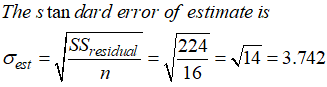#### Earn Coins

Coins can be redeemed for fabulous gifts.

Similar Homework Help Questions
• ### 19. (-/1 Points) DETAILS PRIVITERASTATS3 16.E.023. MY NOTES PRACTICE ANOTHER A researcher tested whether time of...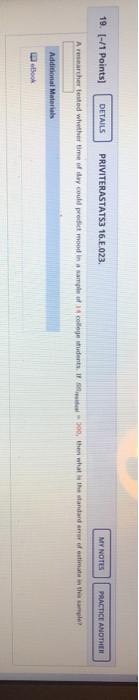19. (-/1 Points) DETAILS PRIVITERASTATS3 16.E.023. MY NOTES PRACTICE ANOTHER A researcher tested whether time of day could predict mood in a sample of 14 college Mudents, 11-100, then what is the standard error of estimate in this sample Additional Materials eBook

• ### +-/2 points PriviteraStats3 16.E.021 My Notes 18 Ask Your Teacher An instructor measured quiz scores and the number...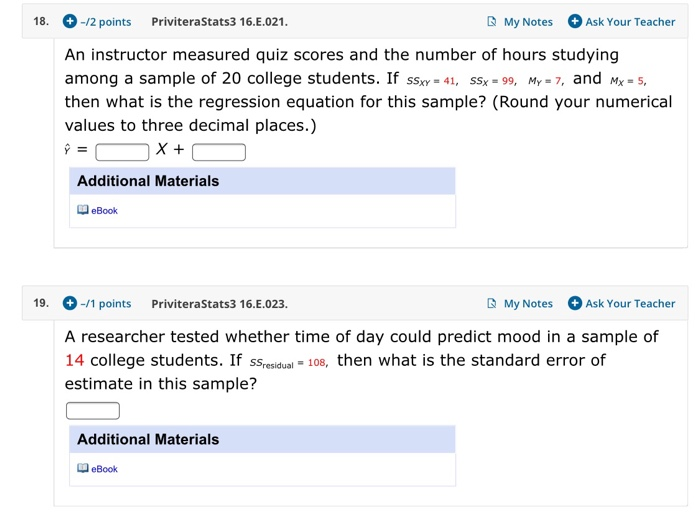+-/2 points PriviteraStats3 16.E.021 My Notes 18 Ask Your Teacher An instructor measured quiz scores and the number of hours studying among a sample of 20 college students. If sSxy 41, SSx 99, My7, and M5, then what is the regression equation for this sample? (Round your numerical values to three decimal places.) Additional Materials eBook Privite ra Stats3 16.E.023. My Notes 19. -/1 points Ask Your Teacher A researcher tested whether time of day could predict mood in a...

• ### A random sample of 46 college students reported the number of hours per day they typically...

A random sample of 46 college students reported the number of hours per day they typically spend on social media. Their sample mean is, M = 2.61, and their standard deviation is, s = 1.02. What is the point estimate of the mean time spent per day on social media for the population of college students?

• ### A researcher examined whether the time of day someone exercises affects memory retention in college courses.  Participants...

A researcher examined whether the time of day someone exercises affects memory retention in college courses.  Participants were assigned to one of three exercise groups: morning, afternoon, evening, and their performance on a memorization task was measured.  This data is below: Morning Afternoon Evening 6 4 7 7 5 6 8 6 8 5 4 6 6 5 5 Mean = 6.40 Mean = 4.80 Mean = 6.40 s = 1.14 s =.84 s = 1.14 Assuming the researcher wants to know...

• ### Students at Hope College were tested to see if they could determine the difference between tap...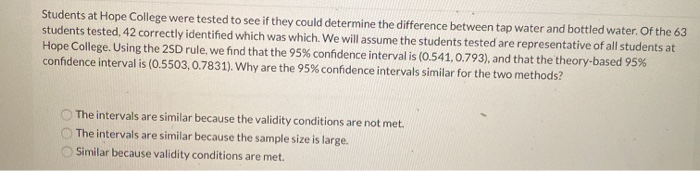Students at Hope College were tested to see if they could determine the difference between tap water and bottled water. Of the 63 students tested, 42 correctly identified which was which. We will assume the students tested are representative of all students at Hope College. Using the 2SD rule, we find that the 95% confidence interval is (0.541,0.793), and that the theory-based 95% confidence interval is (0.5503, 0.7831). Why are the 95% confidence intervals similar for the two methods? The...

• ### A random sample of 46 college students reported the number of hours per day they typically...

A random sample of 46 college students reported the number of hours per day they typically spend on social media. Their sample mean is, M = 2.61, and their standard deviation is, s = 1.02. What is the margin of error, (tci)(SEMs), for this data?

• ### Students at Hope College were tested to see if they could determine the difference between tap...

Students at Hope College were tested to see if they could determine the difference between tap water and bottled water. Of the 63 students tested, 42 correctly identified which was which. We will assume the students tested are representative of all students at Hope College. Using the 2SD rule, we find that the 95% confidence interval is (0.541, 0.793), and that the theory-based 95% confidence interval is (0.5503, 0.7831). Why are the 95% confidence intervals similar for the two methods?...

• ### 2. Suppose that a random sample of 41 state college students is asked to measure the...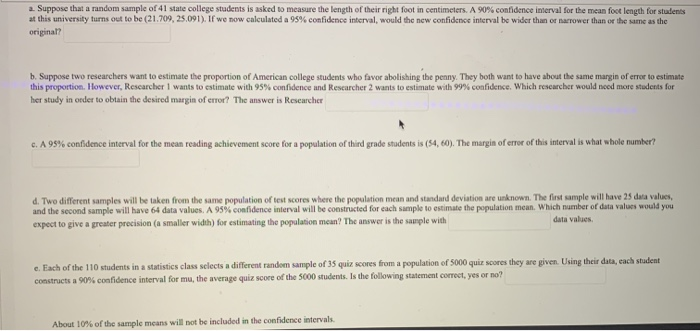2. Suppose that a random sample of 41 state college students is asked to measure the length of their right foot in centimeters. A 90% confidence interval for the mean foot length for students at this university turns out to be (21.709, 25.091). If we now calculated a 95% confidence interval, would the new confidence interval be wider than or narrower than or the same as the original? b. Suppose two researchers want to estimate the proportion of American college...

• ### Suppose that a random sample of 100 part-time college students is 68% female. In this activity,...

Suppose that a random sample of 100 part-time college students is 68% female. In this activity, we calculate the 95% confidence interval for the proportion of all part-time college students that are female.   Recall that the 95% confidence interval is: sample proportion ± 2(SE) where SE is the standard error (or standard deviation). question 2: State the confidence interval. Then convert the values to percentages and interpret the confidence interval in context.

• ### A health researcher does a study investigating the effect of exercise on stress levels in college...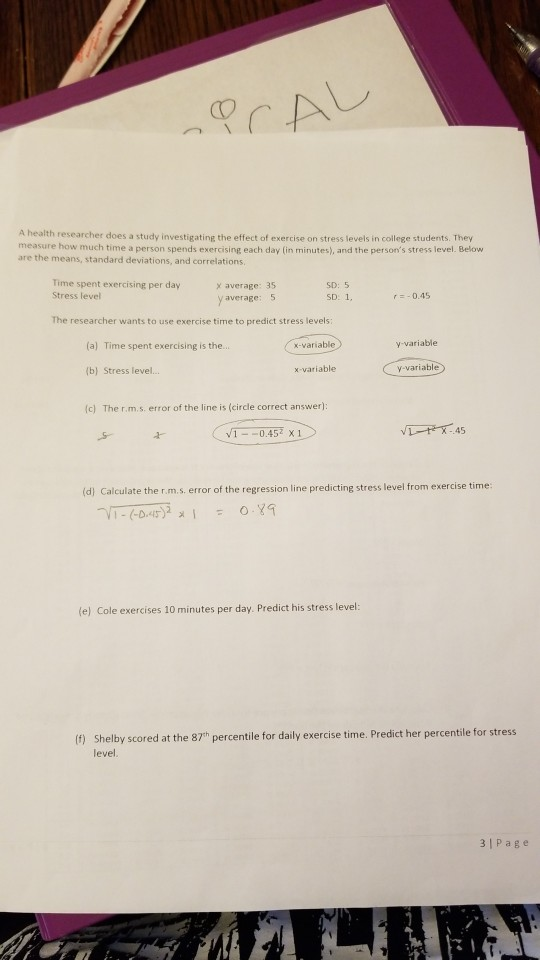A health researcher does a study investigating the effect of exercise on stress levels in college students. Thery measure how much time a person spends exercising each day (in minutes), and the person's stress level. Below are the means, standard deviations, and correlations Time spent exercising per day Stress level x average: 35 average: 5 SD: 5 SD: 1, r -0.45 The researcher wants to use exercise time to predict stress levels (a) Time spent exercising is the.. x-variable y-variable...